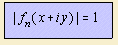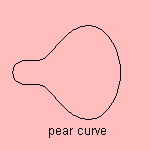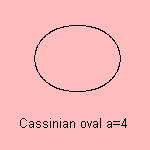# Mandelbrot lemniscate

## higher

last updated: 2004-05-21where fn is defined by the quadratic recurrence relation fn+1(z) = fn2(z) + z with initial f0(z) = 0.

These curves are called equipotential curves by Peitgen and Saupe (1988).
In general, the curve is a polynomial in x and y of degree 2n.

The first three Mandelbrot lemniscates are:

• n = 1: circle
because for the unity circle holds that | f1(z) | = | z | = 1• n = 2: Cassinian oval
| f2(z) | = | z2+z | = 1 leads to a Cassinian oval (a=4)  1).

• n = 3: pear curve
Although the resembles more a bulb, the curve has been given the name of the pear curve.

When n goes to infinity, the curve is the Mandelbrot set.

notes

1) We can write |z2+z | = |z| |z+1| =1 as (x2+y2)((x+1)2+y2) =1. Converting this into the Cartesian form of the Cassinian oval leads to: ((x-1)2+y2)((x+1)2+y2) =42.